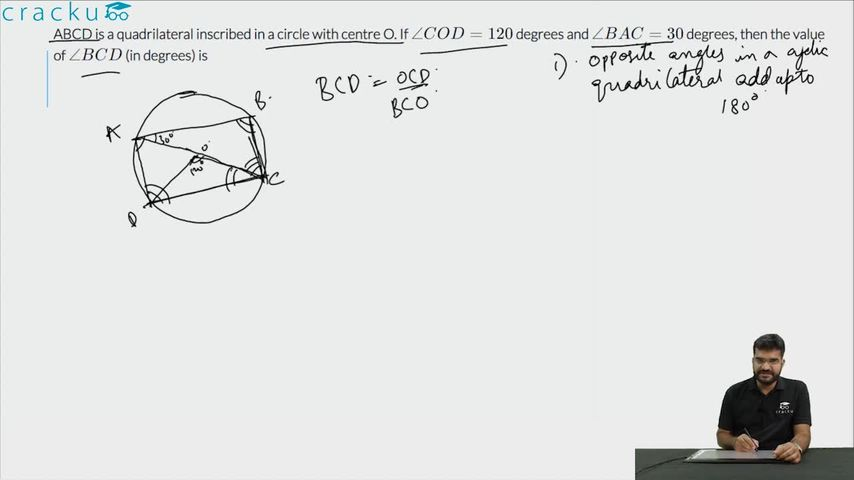Question 84

# ABCD is a quadrilateral inscribed in a circle with centre O such that O lies inside the quadrilateral. If $$\angle COD = 120$$ degrees and $$\angle BAC = 30$$ degrees, then the value of $$\angle BCD$$ (in degrees) is

Solution

$$\angle COD = 120$$ => $$\angle CAD = 120/2 = 60$$ (The angle subtended by the chord DC at the major arc is half the angle subtended at the centre of the circle.)
$$\angle BAC = 30$$
$$\angle BAD = \angle BAC + \angle CAD$$ = 30 + 60 = 90.
$$\angle BCD = 180 - \angle BAD$$ = 180 - 90 = 90

### View Video Solution• All Quant CAT Formulas and shortcuts PDF
• 30+ CAT previous papers with solutions PDF